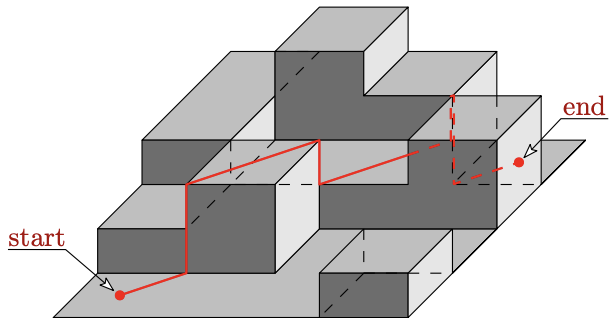시간 제한메모리 제한제출정답맞은 사람정답 비율
2 초 1024 MB104440.000%

## 문제

Escaping from the police over city roofs is often tricky and the gangsters have to be trained properly. To keep up with current AI trends in criminality, they are developing a general computerized model of escape paths.

In the model, the city area where the escape happens is modeled as a 3D grid made of rectangular cuboids with square bases forming a 2D grid on flat ground. Each cuboid represents a block of houses. Top face of a cuboid is called a roof. In the model, all distances between adjacent blocks are reduced to 0. The path of escaping gangsters is modeled as a polyline – a sequence of straight horizontal and vertical segments where the end point of one segment is the start point of the next segment. The basic path properties are:

• Each point on the path is on the surface of at least one block.
• No part of the path is in the interior of any block.
• The height of any point on the path is bigger than or equal to the lowest height of roofs of all blocks to which surface the point belongs.
• The path starts and ends in the center of a block roof.
• The sum over the lengths of horizontal segments of the path is minimum possible.

It may happen that two consecutive segments on the path share common points. This stems from the fact that the path models a real behavior of a person moving over physical obstacles. Thus an additional path rule also holds:

• Let P be a point on the path. If there is a point Q directly above P, and Q belongs to at least two blocks, then the point Q is on the path.

The total length of the escape path should be carefully calculated in the model.## 입력

The first line of the input contains six positive integers W, H, Sx, Sy, Ex, Ey (1 ≤ W · H ≤ 105, 1 ≤ Sx, Ex ≤ W, 1 ≤ Sy, Ey ≤ H). W and H are even integers representing the dimensions of the grid base in meters, integers Sx, Sy denote starting coordinates of the escape path and Ex, Ey denote coordinates of the end.

Each of the next H/2 lines contains W/2 integers, the i-th integer on j-th line is the height of the corresponding block Ti,j in meters (0 ≤ Ti,j ≤ 103). Each grid block base is a square with dimensions of 2 × 2 meters in the model.

## 출력

Print the length of the escape path. The difference between the printed length and the exact length must be less than 10−4.

## 예제 입력 1

8 8 1 7 7 1
2 3 2 0
2 1 1 2
1 2 0 0
0 0 0 1


## 예제 출력 1

14.485281374238


## 힌트

The sample input with its solution is shown on the picture above.